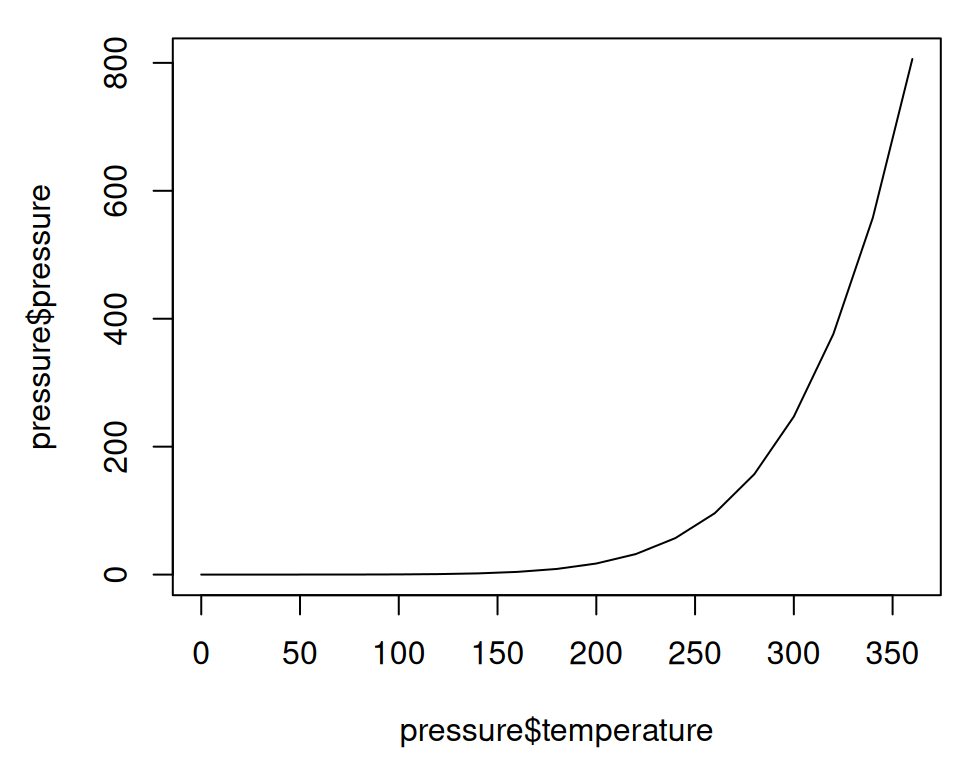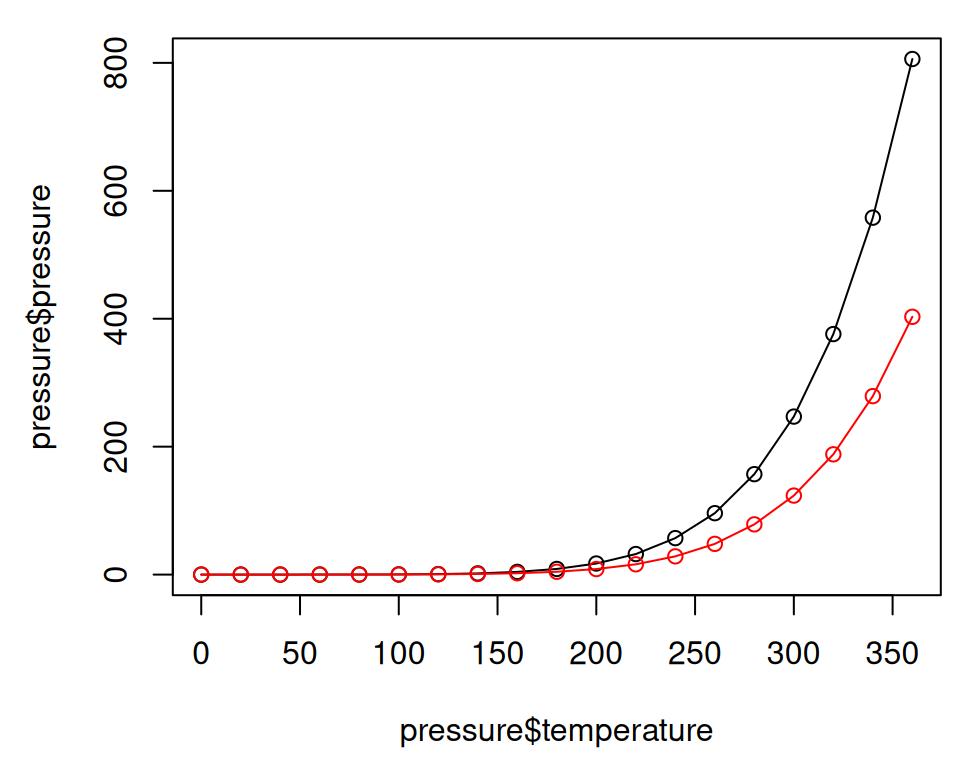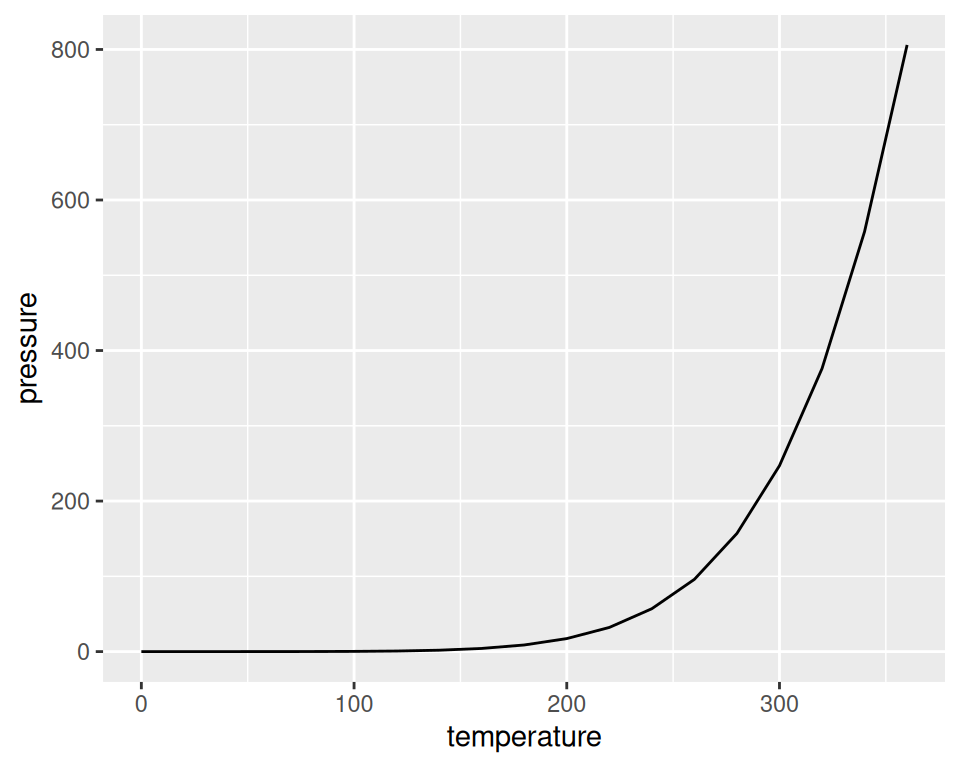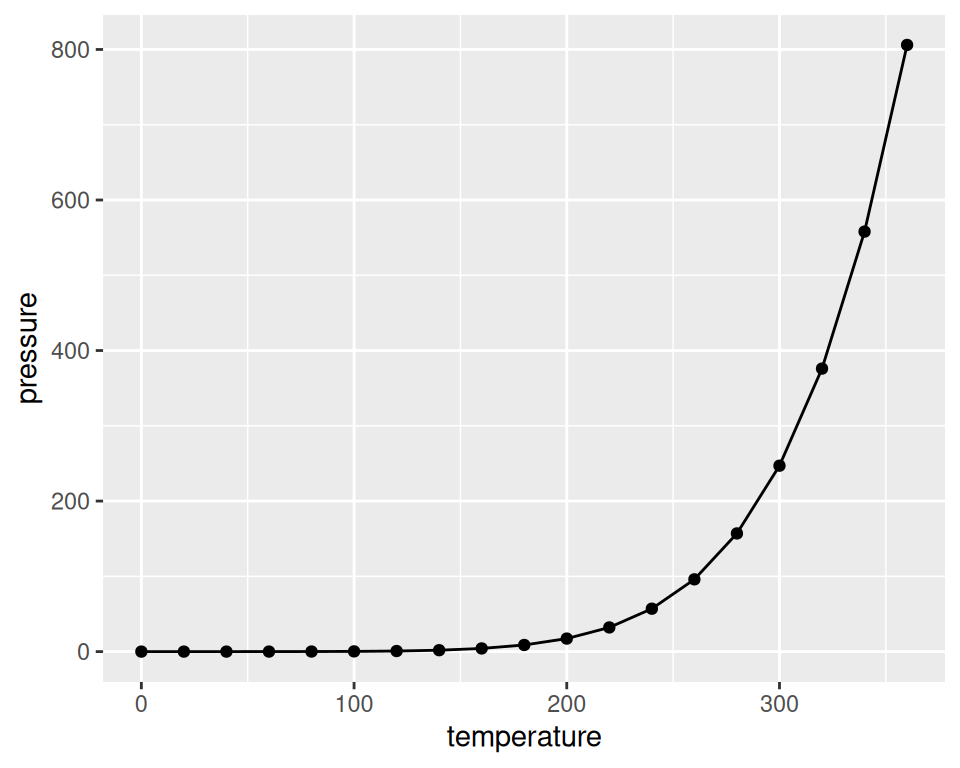## 2.2 Creating a Line Graph

### 2.2.1 Problem

You want to create a line graph.

### 2.2.2 Solution

To make a line graph using `plot()` (Figure 2.3, left), pass it a vector of x values and a vector of y values, and use `type = "l"`:

``plot(pressure\$temperature, pressure\$pressure, type = "l")``Figure 2.3: Line graph with base graphics (left); With points and another line (right)

To add points and/or multiple lines (Figure 2.3, right), first call `plot()` for the first line, then add points with `points()` and additional lines with `lines()`:

``````plot(pressure\$temperature, pressure\$pressure, type = "l")
points(pressure\$temperature, pressure\$pressure)

lines(pressure\$temperature, pressure\$pressure/2, col = "red")
points(pressure\$temperature, pressure\$pressure/2, col = "red")``````

With ggplot2, you can get a similar result using `geom_line()` (Figure 2.4):

``````library(ggplot2)
ggplot(pressure, aes(x = temperature, y = pressure)) +
geom_line()``````Figure 2.4: Line graph with `ggplot()` (left); With points added (right)

As with scatter plots, you can pass you data in vectors instead of in a data frame (but this will limit the things you can do later with the plot):

``````ggplot(pressure, aes(x = temperature, y = pressure)) +
geom_line() +
geom_point()``````

Note

It’s common with `ggplot()` to split the command on multiple lines, ending each line with a `+` so that R knows that the command will continue on the next line.